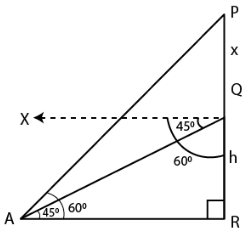Guru

# A pole of height 5 m is fixed on the top of a tower. The angle of elevation of the top of the pole as observed from a point A on the ground is 60 and the angle of depression of the point A from the top of the tower is 45. Find the height of the tower. (Take √3 = 1.732)

• 1

sir this is the important  question from the book -ML aggarwal( avichal publication) class 10th , chapter20 , heights and distances
this question has been asked in previous year exams
it is given that A pole of height 5 m is fixed on the top of a tower. The angle of elevation of the top of the pole as observed from a point A on the ground is 60 and the angle of depression of the point A from the top of the tower is 45. Find the height of the tower. (Take √3 = 1.732)

question no 33 , heights and distances , ICSE board, ML Aggarwal

Share

1. Consider QR as the tower

PQ as the pole on it

Angle of elevation from P to a point A is ∠PAR = 600

Angle of depression from Q to A = 450

Here

∠QAR = 450 which is the alternate angle

PQ = 5 m

Take QR = h m

PQ = 5 + hIn right triangle QAR

tan θ = QR/AR

Substituting the values

tan 450 = h/AR

So we get

1 = h/AR

AR = h

In right triangle PAR

tan 600 = PR/AR

Substituting the values

√3 = (5 + h)/ h

So we get

√3h = 5 + h

h (√3 – 1) = 5

h (1.732 – 1) = 5

By further calculation

0.732 h = 5

h = 5/0.732 = 5000/732

h = 6.83

Hence, the height of the tower is 6.83 m.

• 1